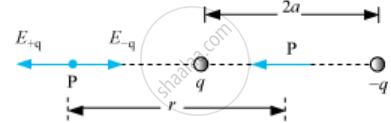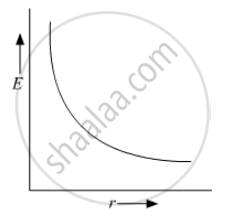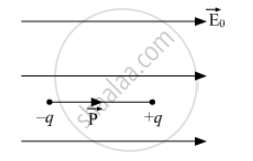PUC Karnataka Science Class 12Department of Pre-University Education, Karnataka
Share

# Derive an expression for the electric field E due to a dipole of length '2a' at a point distant r from the centre of the dipole on the axial line - PUC Karnataka Science Class 12 - Physics

#### Question

(a) Derive an expression for the electric field E due to a dipole of length '2a' at a point distant r from the centre of the dipole on the axial line.
(b) Draw a graph of E versus r for r >> a.
(c) If this dipole were kept in a uniform external electric field E0, diagrammatically represent the position of the dipole in stable and unstable equilibrium and write the expressions for the torque acting on the dipole in both the cases.

#### Solution

(a) Electric Field on Axial Line of an Electric Dipole:Let P be at distance r from the centre of the dipole on the side of charge q. Then,

E_(-q)=q/(4piepsilon_0(r+a)^2)hatp

Where, hatp is the unit vector along the dipole axis (from − q to q). Also,

E_(+q)=q/(4piepsilon_0(r-a)^2)hatp

The total field at P is

E=E_(+q)+E_(-q)=q/(4piepsilon_0)[1/(r-a)^2-1/(r+a)^2]hatp

E=q/(4piepsilon_0)(4ar)/(r^2-a^2)^2hatp

for r >> a

E=(4qa)/(4piepsilon_0r^3)hatp   (r >> a)

E=(2p)/(4piepsilon_0r^3)  [because vecp=qxxvec(2a)hatp]

(b) For r >> a, E prop 1/r^3(c) Position of dipole in stable equilibrium: In stable equilibrium, dipole aligns itself in the direction of external electric field.Therefore, angle (θ) between vecP and vecE_0 is 0 and we know torque acting on the dipole in an external field is given by the expression
τ = PEsinθ

As, θ is 0° for stable equilibrium

Therefore, τ = PEsinθ

τ= PEsin0°

τ = 0

Position of dipole in unstable equilibrium: In unstable equilibrium, dipole aligns itself in the direction opposite to the direction of external electric field.τ = PEsinθ

As, θ is 180° for unstable equilibrium

Therefore, τ = PEsinθ

τ= PEsin180°

τ = 0

Is there an error in this question or solution?

#### Video TutorialsVIEW ALL 

Solution Derive an expression for the electric field E due to a dipole of length '2a' at a point distant r from the centre of the dipole on the axial line Concept: Electric Field Lines.
S